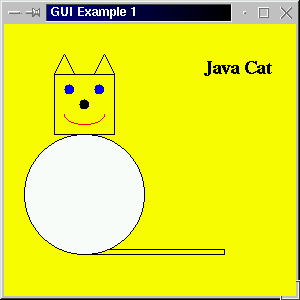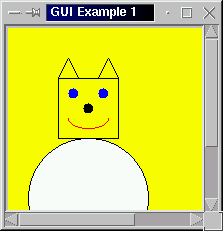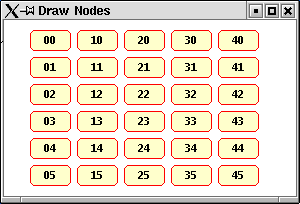# GRAPHICS

### CONTENTS

NOTE: These examples were written some time ago (April 2000), all use the java.awt classes; much more versatile graphical capabilities can be found in the Java2D classes. There are some examples of the use of these classes given in this collection of www pages.

## 1. INTRODUCTION

The awt class Component contains a method paint which is automatically invoked whenever a component is drawn or redrawn. This is inheritted by all sub-classes of the class Component. The awt package also conatins a component canvas which is an area in which we can draw using methods found in the Graphics class. A canvas is the same as any other component and can be placed in a container as desired using the Canvas constructor. When the container is made vissible this will then autonatically invoke the paint method.

If we wish to include a drawing in our Canvas component we must overide the paint method by extending the canvas class with our own class which includes our own paint method.

## 2. EXAMPLE

In the code presented in Table 1 we creare a class MyCanvas which extends the class Canvas and includes our own version of the paint method contained in the Component class. The paint method comprises a sequance of methods taken from the Graphics class which will produce Skansholm's cat (Skansholm 2000). We then create a class Gui that extends the class Frame and implements the interface WindowListener. We also include an application class GraphicsEx. The output from the code is presented in Figure 1.

 ```// GRAPHICS EXAMPLE // Frans Coenen // MTuesday 2 April 2000 // Dept. of Comp. Sci., University of Liverpool import java.awt.event.*; import java.awt.*; class MyCanvas extends Canvas { public void paint(Graphics g) { g.setColor(Color.black); g.drawRect(50,50,60,60); // Head g.drawRect(80,225,140,5); // Tail g.setColor(Color.white); g.fillOval(20,110,120,120); // White body g.setColor(Color.black); g.drawOval(20,110,120,120); // Outline of body g.fillOval(75,75,10,10); // Nose g.setColor(Color.blue); g.fillOval(60,60,10,10); // Eyes g.fillOval(90,60,10,10); g.setColor(Color.black); g.drawLine(50,50,60,30); // ears g.drawLine(60,30,70,50); g.drawLine(110,50,100,30); g.drawLine(100,30,90,50); g.setColor(Color.red); g.drawArc(60,80,40,20,180,180); // Mouth g.setColor(Color.black); Font serif = new Font("Serif",Font.BOLD,18); g.setFont(serif); g.drawString("Java Cat",200,50); } } class Gui extends Frame implements WindowListener { Graphics pic; /* Constructor */ public Gui(String text) { super(text); setBackground(Color.yellow); addWindowListener(this); MyCanvas pic = new MyCanvas(); add(pic); } /* Window Closed */ public void windowClosed(WindowEvent event) { } /* Window Deiconified */ public void windowDeiconified(WindowEvent event) { } /* Window Iconified */ public void windowIconified(WindowEvent event) { } /* Window Activated */ public void windowActivated(WindowEvent event) { } /* Window Deactivated */ public void windowDeactivated(WindowEvent event) { } /* Window Opened */ public void windowOpened(WindowEvent event) { } /* Window Closing */ public void windowClosing(WindowEvent event) { System.exit(0); } } class GraphicsEx { /* Main method */ public static void main(String[] args) { Gui screen = new Gui("GUI Example 1"); screen.setSize(300,300); screen.setVisible(true); } } ```

Table 1:Code to produce output given in Figure 1Figure 1: Skansholm's cat (Skansholm 2000) produced by exacuting code presented in Table 1.

The Graphics class contains many other methods.For example:

1. clearRect(int x, int y, int width, int height) clears the specified rectangle by filling it with the background colour of the current drawing surface.

## 3. SCROLL PANES

Sometimes a picture is too big to fit neatly into a window. In sucvh a case we like to be able to display part of it and be able to scroll across the picture to reveal other parts. We do this by creating a scroll pane. A scroll pane object is a container that can only hold a single component (e.g. a canvas). We can rewite the Gui constructor given in Table 1 as shown in Table 2 to include a scroll pane.

 ```public Gui(String text) { super(text); addWindowListener(this); // Create scroll pane ScrollPane sp1 = new ScrollPane(); add(sp1); // Create canvas MyCanvas pic = new MyCanvas(); pic.setBackground(Color.yellow); sp1.add(pic); } ```

Table 2:Including a scroll pane in the example given in Table 1Figure 2: Example output with scroll pane

## 4. WORKING WITH PARAMETERS

In the cat drawing example we simply drew an image, for many applications (e.g. graph drawing), we nee to be able to pass parameters to our drawing methods. To do this it makes sence to split the drawing classes (e.g. the class GraphicsEx) into an application class and a drawing class. For example if we wish to create a program that draws a line given a pair of start coordinates and a pair of end coordinates we might create two classes as shown in Tables 3 and 4.

The class in Table 3 contains all the methods necessary to draw the desired image starting with a single top level method which can be called from the user's self define paint method contained in some extension of the built in class Canvas. The class also has a number of attributes to which values can be assigned using the class' constructor --- these are then the parameters for the image.

 ```// LINE DRAWING PROGRAM // Frans Coenen // 26 June 2000 // Department of Computer Science, University of Liverpool import java.awt.*; class DrawLine { // Variables for graph drawing private int xStart, yStart; private int xEnd, yEnd; /* Constructor */ public DrawLine(int x1, int y1, int x2, int y2) { xStart = x1; yStart = y1; xEnd = x2; yEnd = y2; } /* Draw the line */ public void outputLine(Graphics g) { g.drawLine(xStart,yStart,xEnd,yEnd); } } ```

Table 4: Drawing class

The class in Table 3 is the application class which contains the main method. This then creates an instance of the drawing class (DrawLine --- Table 4) in such a way that values are passed to the drawing class. The main method also creates an instance of the class Gui (Table 5) paassing the drawing class instance name at the same time.

 ```// DRAW LINE APPLICATION PROGRAM // Frans Coenen // 26 June 2000 // Department of Computer Science, University of Liverpool import java.io.*; import DrawLine; class DrawLineApp { // ------------------- FIELDS ------------------------ // Create BufferedReader class instance public static InputStreamReader input = new InputStreamReader(System.in); public static BufferedReader keyboardInput = new BufferedReader(input); // Sizes private static final int WIDTH = 500; // Total Width private static final int HEIGHT = 400; // Total height // ------------------ METHODS ------------------------ /* MAIN: Main method */ public static void main(String[] args) throws IOException { int x1, y1, x2, y2; // Input x and y for start of line System.out.println("Input x and y for start of line (integers)"); x1 = new Integer(keyboardInput.readLine()).intValue(); y1 = new Integer(keyboardInput.readLine()).intValue(); // Input x and y for point 2 System.out.println("Input x and y for end of line (integers)"); x2 = new Integer(keyboardInput.readLine()).intValue(); y2 = new Integer(keyboardInput.readLine()).intValue(); // Create an instance of the class DrawLine DrawLine myLine = new DrawLine(x1,y1,x2,y2); // Draw line Gui screen = new Gui("Draw Line",myLine,WIDTH,HEIGHT); screen.setSize(WIDTH,HEIGHT); screen.setVisible(true); } } ```

Table 4: Application class

Table 5 shows the class Gui which is similar to the class with the same name given in Table 1. The difference is only that the second argument of the constructor is an instance of the class DrawLine which is passed as a parameter when an instance of the class myCanvas is created.

 ```// GUI // Frans Coenen // 6 April 2000 // Department of Computer Science import java.awt.event.*; import java.awt.*; class Gui extends Frame implements WindowListener { /* Constructor */ public Gui(String text, DrawLine myLine, int width, int height) { super(text); addWindowListener(this); // Scroll pane ScrollPane sp1 = new ScrollPane(); add(sp1); // Canvas MyCanvas pic = new MyCanvas(myLine); pic.setSize(width-50,height-50); pic.setBackground(Color.white); sp1.add(pic); } /* Window Closed */ /* Window Deiconified */ /* Window Iconified */ /* Window Activated */ /* Window Deactivated */ /* Window Opened */ /* Window Closing */ } ```

Table 5: GUI class

Table 6 shows the version of the myCanvas class required here. Note that it has a field DrawLine which an isnatnce of the drawing class presented in Table 4. Note also that the top level drawing method contained in the drawing class is called from within the paint method.

 ```// MY CANVAS // Frans Coenen // Tuesday 2 April 2000 // Dept. of Comp. Sci., University of Liverpool import java.awt.event.*; import java.awt.*; class MyCanvas extends Canvas { private DrawLine myLine; public MyCanvas(DrawLine line) { super(); myLine = line; } public void paint(Graphics g) { myLine.outputLine(g); } } ```

Table 6: Extension to Canvas class

## 5. DRAWING ROUNDED RECTAQNGLES (SWING EXAMPLE)

The code in Table 1 gives an example of drawing rounded rectangles, the resulting output is given in Figure 1.

 ```// JAVA NODES // Frans Coenen // MOnday 30 June 2003 // The University of Liverpool, UK /* Based on example given by Deitel and Deital */ import java.awt.*; import java.awt.event.*; import java.awt.geom.*; import java.awt.image.*; // Java extension packages import javax.swing.*; public class Node extends JFrame { // ------------------- FIELDS ------------------------ /* CONSTANTS */ static final int NODE_WIDTH = 40; static final int NODE_HEIGHT = 20; static final int ARC_WIDTH = 10; static final int ARC_HEIGHT = 10; // ------------------ CONSTRUCTORS ------------------- public Node() { super("Draw Nodes"); getContentPane().setBackground(Color.white); setSize(300,200); setVisible(true); } // ------------------ METHODS ------------------------ /* PAINT */ /** Draws general paths. */ public void paint(Graphics g) { // Call superclass paint method super.paint(g); for(int index1=0;index1<5;index1++) { for(int index2=0;index2<6;index2++) { drawNode(g,30+(index1*(NODE_WIDTH+7)),30+(index2*(NODE_HEIGHT+7)), Integer.toString(index1)+Integer.toString(index2)); } } } public void drawNode(Graphics g, int x, int y, String label) { g.setColor(new Color(255,255,204)); g.fillRoundRect(x,y,NODE_WIDTH,NODE_HEIGHT,ARC_WIDTH,ARC_HEIGHT); g.setColor(Color.red); g.drawRoundRect(x,y,NODE_WIDTH,NODE_HEIGHT,ARC_WIDTH,ARC_HEIGHT); // Lavel g.setColor(Color.black); Font mono = new Font("Monospaced",Font.BOLD,10); g.setFont(mono); FontMetrics newFM = g.getFontMetrics(mono); int xLength = newFM.stringWidth(label); g.drawString(label,(x+(NODE_WIDTH/2)-(xLength/2)),y+14); } /* MAIN METHOD */ public static void main(String args[]) { Node application = new Node(); application.setDefaultCloseOperation(JFrame.EXIT_ON_CLOSE); } } ```

Table 7: Drawing rounded rectanglesFigure 3 :Output from code presented in Table 7

## 5. REFERENCES

1. Skansholm, J. (2000). Java from the Beginning. Addison-Wesley, London, p23.

Created and maintained by Frans Coenen. Last updated 30 June 2003